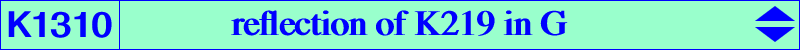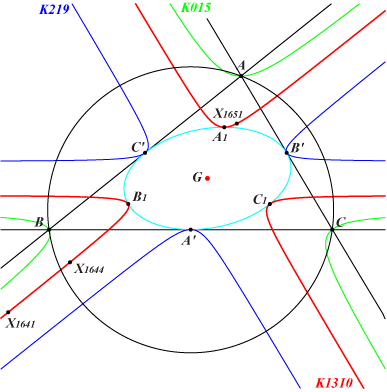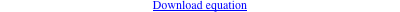too complicated to be written here. Click on the link to download a text file.X(2), X(1641), X(1644), X(1651), X(8371), X(14474), X(14475), X(14476), X(14477), X(36847) X(52720) → X(52724) infinite points of the sidelines of ABC A1, B1 C1 : midpoints of AG, BG, CG on the Steiner inellipse homothetic images of B, C under h(A',1/√3) with A' = midpoint of BC, and other points cyclically. These six points lie on the homothetic of the Steiner ellipse under h(G,1/√2) Geometric properties :K1310 is the locus of tripolar centroid of points on the Steiner ellipse. K1310 is an acnodal cubic with singularity at G. The imaginary nodal tangents at G pass through the infinite points of the Steiner ellipse. K1310 is the reflection of K219 in G. Recall that K219 is the locus of tripolar centroid of points on the line at infinity. K1310 is also the homothetic image of K015 under h(G,1/2), of K656 under h(G,1/3), of K700 under h(G,-2/3). See also CL045. Other simple tripolar centroids on K1310 : a^2 (b-c) (b+c) (a^4 b^4-4 a^4 b^2 c^2+2 a^2 b^4 c^2+a^4 c^4+2 a^2 b^2 c^4-2 b^4 c^4) : : . (-a^4 b^4-a^4 c^4+2 b^4 c^4) (-a^8 b^8+4 a^8 b^4 c^4-2 a^4 b^8 c^4-a^8 c^8-2 a^4 b^4 c^8+2 b^8 c^8) : : . a^4 (b-c) (b+c) (b^2+c^2) (a^8 b^8-4 a^8 b^4 c^4+2 a^4 b^8 c^4+a^8 c^8+2 a^4 b^4 c^8-2 b^8 c^8) : : . a^3 (b-c) (b^2+b c+c^2) (a^6 b^6-4 a^6 b^3 c^3+2 a^3 b^6 c^3+a^6 c^6+2 a^3 b^3 c^6-2 b^6 c^6) : : . (b-c) (b^2+b c+c^2) (-2 a^6+2 a^3 b^3+b^6+2 a^3 c^3-4 b^3 c^3+c^6) : : . (b-c) (b+c) (b^2+c^2) (-2 a^8+2 a^4 b^4+b^8+2 a^4 c^4-4 b^4 c^4+c^8) : : . (2 a^3-b^3-c^3) (2 a^6-2 a^3 b^3-b^6-2 a^3 c^3+4 b^3 c^3-c^6) : : . (2 a^4-b^4-c^4) (2 a^8-2 a^4 b^4-b^8-2 a^4 c^4+4 b^4 c^4-c^8) : : .